# WTMA Sample Questions

This test asks questions about everyday objects, things you might find in the kitchen or in other places in the home, or as you go about your everyday life.

None of these sample questions are from the test.

## Sample Questions

Sample Question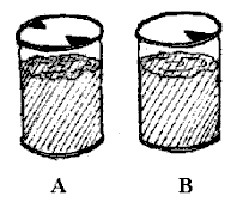Look at this drawing of two cans of juice. Which will pour more easily?

(A) Can A

(B) Can B

(C) No difference

Sample Question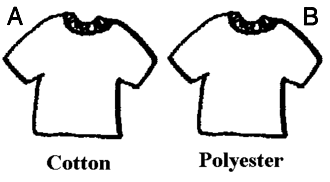Which of these new shirts is more likely to shrink if washed in hot water?

(A) A

(B) B

(C) Can't tell

Sample Question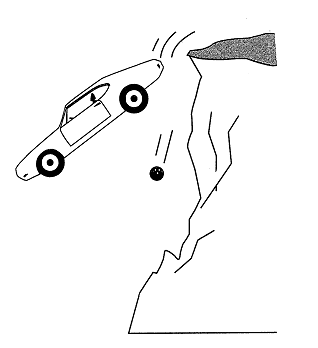If a car and a bowling ball were thrown off a 100 foot cliff at the same time, which would hit the ground first?

(A) Car

(B) Ball

(C) No difference

Sample Question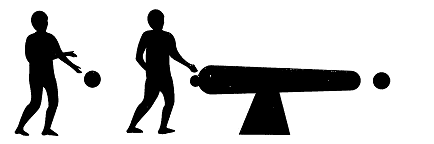If a cannon fires a cannonball horizontally and you drop a cannonball at the same time, which will hit the ground first?

(A) Drop

(B) Fire

(C) No difference

Sample QuestionYou tie a string to the ceiling and attach a weight to the end. You hold the weight next to your face but not touching it and then let go. The weight swings down and away from you and then starts swinging back toward you. If you do not move, will it hit you?

(A) Yes

(B) No

(C) Can't tell

Sample Question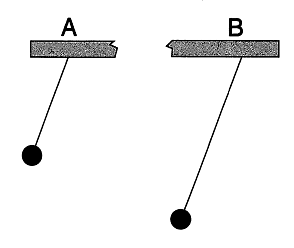You put the same amount of weight at the end of these two pendulums. If you let go of the two pendulums at the same time, which will swing back and forth more times in a minute?

(A) A

(B) B

(C) No difference

Sample QuestionThese children weigh the same. Will this seesaw balance?

(A) Yes

(B) No

(C) Can't tell

Sample QuestionWhich of these pairs of magnets will stick together in the positions they are in?

(A) Drawing A

(B) Drawing B

(C) Both Drawings

Sample Question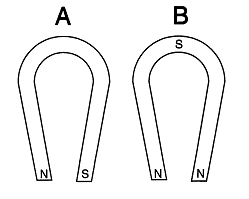Which of these show how the poles are arranged on horseshoe magnets?

(A) Drawing A

(B) Drawing B

(C) Neither

Sample QuestionWhich ball would bounce higher if dropped from the same height?

(A) Ball A

(B) Ball B

(C) No difference

Sample Question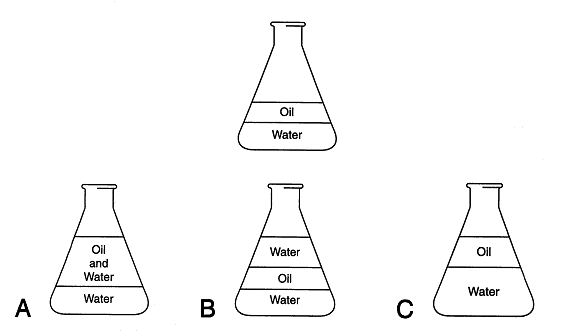At the top is a drawing of a glass container holding water and oil. If you add more water what would it look like?

(A) A

(B) B

(C) C

Sample QuestionThese stools are the same height. Which is more likely to tip over when an active child sits on it?

(A) Stool A

(B) Stool B

(C) No difference

Sample QuestionIf the shopping cart was moving in the direction of the big arrow and then you stopped it suddenly, which way would the milk carton fall?

(A) A

(B) B

(C) Can't tell

Sample Question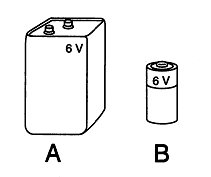Which battery has the higher voltage?

(A) Battery A

(B) Battery B

(C) There's no difference

Sample QuestionWhich wheels are turning in the same direction as wheel 4?

(A) 1, 2, and 3

(B) 1, 2, and 5

(C) 1, 3, and 5

Sample Question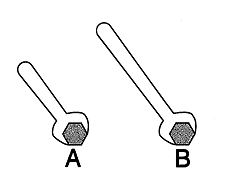Which wrench will make it easier to tighten the bolt?

(A) A

(B) B

(C) There's no difference

Sample Question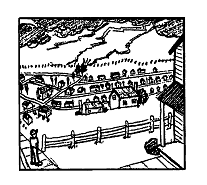Lightning strikes at one end of a city. You are at the other end of the city. Would you hear the thunder or see the lightning first?

(A) Hear the thunder

(B) See the lightning

(C) You will hear and see at the same time

Sample Question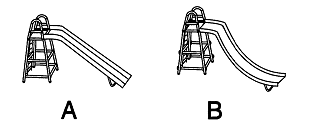Which slide will give the faster ride?

(A) A

(B) B

(C) There's no difference

Sample QuestionWill this bulb light up?

(A) Yes

(B) No

(C) Can't tell

Sample QuestionYou want to lift this pole off the ground and carry it using one hand. Where should you pick it up to be able to carry it most easily?

(A) A

(B) B

(C) C

Sample QuestionIn this typical traffic signal, which light is the red one?

(A) A

(B) B

(C) C

Sample Question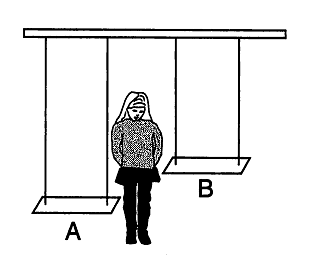The girl wants to swing fast. After she gets up to full speed, on which swing will she cover more distance in 1 minute?

(A) A

(B) B

(C) No difference

Sample Question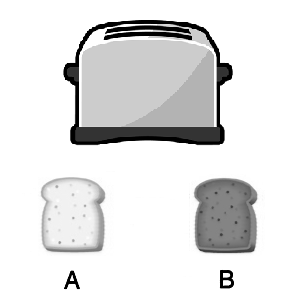You start with two slices of bread that are exactly the same. You toast slice B. Which slice weighs more now?

(A) A

(B) B

(C) There is no difference

Sample Question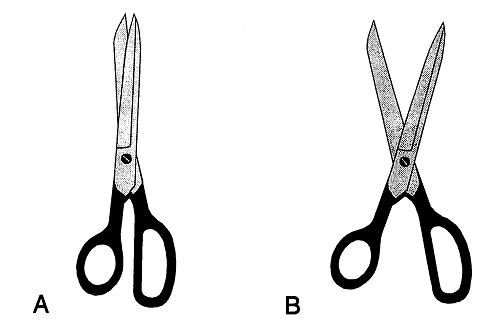How far should you open the scissors so it is easier to cut a piece of cardboard?

(A) A

(B) B

(C) There is no difference

Sample QuestionWhich wheel is turning faster?

(A) A

(B) B

(C) No difference

Sample QuestionWhich button should you push to close the doors of an elevator?

(A) A

(B) B

(C) Can't tell

Sample QuestionWhich light bulb gets hotter?

(A) A

(B) B

(C) Can't tell

Sample QuestionWhich bulb will light up?

(A) Drawing A

(B) Drawing B

(C) Both

Sample Question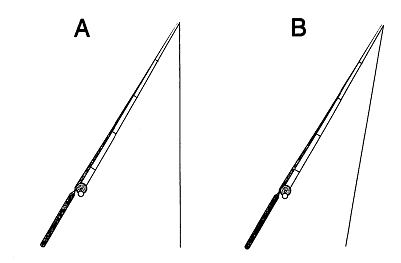Which way will the fishing line hang when the fishing rod is tilted?

(A) Drawing A

(B) Drawing B

(C) Either

Sample QuestionWhich stick is easier to balance on the palm of your hand?

(A) Stick A

(B) Stick B

(C) No difference